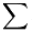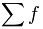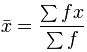# The Mean from a Frequency Table

It is easy to calculate the Mean:

then divide by how many numbers there are.

### Example: What is the Mean of these numbers?

6, 11, 7

• Add the numbers: 6 + 11 + 7 = 24
• Divide by how many numbers (there are 3 numbers): 24 ÷ 3 = 8

### The Mean is 8

But sometimes we don't have a simple list of numbers, it might be a frequency table like this (the "frequency" says how often they occur):

Score Frequency
1 2
2 5
3 4
4 2
5 1

(it says that score 1 occurred 2 times, score 2 occurred 5 times, etc)

We could list all the numbers like this:

Mean = 1+1 + 2+2+2+2+2 + 3+3+3+3 + 4+4 + 5(how many numbers)

But rather than do lots of adds (like 3+3+3+3) it is easier to use multiplication:

Mean = 1 + 2 + 3 + 4 + 5 (how many numbers)

And rather than count how many numbers there are, we can add up the frequencies:

Mean = 1 + 2 + 3 + 4 + 5 2 + 5 + 4 + 2 + 1

And now we calculate:

Mean = 2 + 10 + 12 + 8 + 5 14
=  3714  =  2.64...

And that is how to calculate the mean from a frequency table!

Here is another example:

### Example: Parking Spaces per House in Hampton Street

Isabella went up and down the street to find out how many parking spaces each house has. Here are her results:

Parking
Spaces
Frequency
1 15
2 27
3 8
4 5

What is the mean number of Parking Spaces?

Mean = 15×1 + 27×2 + 3 + 415 + 27 + 8 + 5
15 + 54 + 24 + 2055
2.05...

The Mean is 2.05 (to 2 decimal places)

(much easier than adding all numbers separately!)

## Notation

Now you know how to do it, let's do that last example again, but using formulas.This symbol (called Sigma) means "sum up" (read more at Sigma Notation)

So we can say "add up all frequencies" this way:(where f is frequency)

And we can use it like this:Likewise we can add up "frequency times score" this way:(where f is frequency and x is the matching score)

And the formula for calculating the mean from a frequency table is:The x with the bar on top says "the mean of x"

So now we are ready to do our example above, but with correct notation.

### Example: Calculate the Mean of this Frequency Table

x f
1 15
2 27
3 8
4 5

And here it is:

x = ΣfxΣf = 15×1 + 27×2 + 8×3 + 5×415+27+8+5
= 2.05...

There you go! You can use sigma notation.

## Calculate in the Table

It is often better to do the calculations in the table.

### Example: (continued)

From the previous example, calculate f  × x in the right-hand column and then do totals:

x f fx
1 15 15
2 27 54
3 8 24
4 5 20
TOTALS: 55 113

And the Mean is then easy:

Mean = 11355 = 2.05...